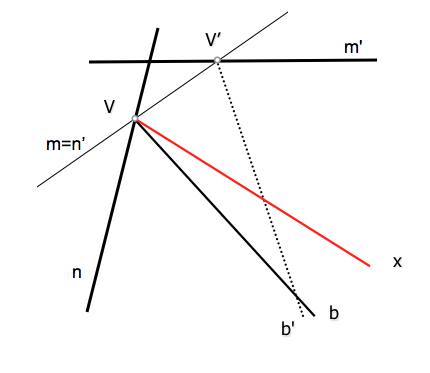# Geometría proyectiva: Determination of homologous elements in projective beams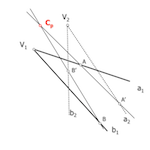One of the first problems we must learn to work in projective geometry is the identifying homologous elements, both series as bundles and any provision of bases, or separate superimposed.

To continue the study of the methodology to be used will use the dual model the elements based on “points”, ie with straight, further assuming that the bases of the respective beams are separated relate.

Therefore we will consider the determination of projective two homologous elements do not have common elements. The problem statement, generally, can be:

Given two projective bundles defined by three pairs of elements (straight) counterparts, determine the counterpart of a given beam.

The lightning data can belong to any of the beams and therefore we seek belong to the base of another.

We will solve this problem by using intermediate perspectividades to establish between the two projective bundles, thereby obtaining the projective center of the two beams (point Cp). As we saw, the projective center of the beams is the perspective center of the series we get to dissect the rays of a beam by an element of any other, and simultaneously severing their counterparts from the counterpart of the geometric element used as a basis in the first section.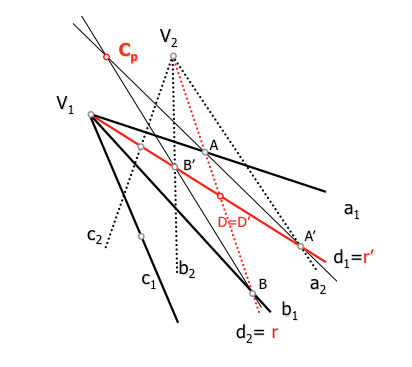Projective Center two beams (Center prospective series)

We will determine in any case, therefore, the projective center two beam.

### Getting the projective center of two beams:

The different cases that may occur will be determined by the data defining projective bundles, can be in principle:

• Pair of ordinary ray counterparts (3 maximum)
• Ordinary ray counterparts to bases ( 2 maximum)
• Locus in which the axis is projective

We can combine these data to determine a specific problem, whenever we bring the necessary number of them. The problem will be determined when we know three pairs of homologous elements or equivalent data. Therefore solve this first case:

Given three straight (ray) of a beam and its homologs, determine the projective center of said beams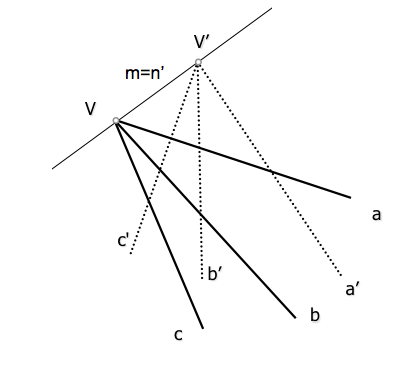The data are the lines to, b y c (ray beam vertex V) as well as their corresponding counterparts rays', b’ y c’. The common beam bases m = n’ contain a line for each of the beams.

To determine the projective center need a couple of lines containing it. These can be determined as the projection of two homologous points in two perspectives series base a pair of homologous rays.

The locus obtained can be considered as a projection beam of two homologous series of points obtained by sectioning by byb’ the c and c rays', but we also understand that the foundation of the series are cyc’ and sectioned beams b and b '.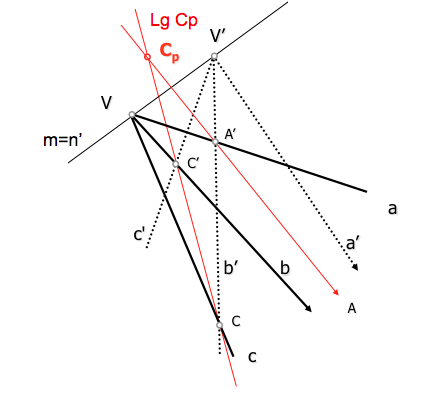The center has been determined by the intersection of the locus which have previously found and another which is obtained similarly to the previous, b rays to relate with their counterparts’ y b’, give the points A and A’ perspectives series.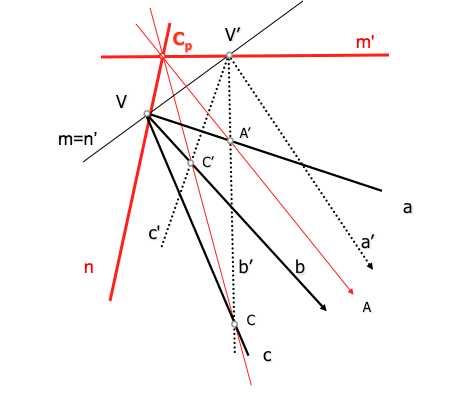Homologous rays containing the bases are the lines that project the projective center from each of the bases (Vertices of the beams). These elements can be obtained as the counterpart of any ray x or and’ unknown.

### Obtaining similar elements

Using the projective center is easy to determine the counterpart of any ray; example we obtain the counterpart of a point X.

To simplify the figure we are left with an element a and its counterpart a'y center projective bundles.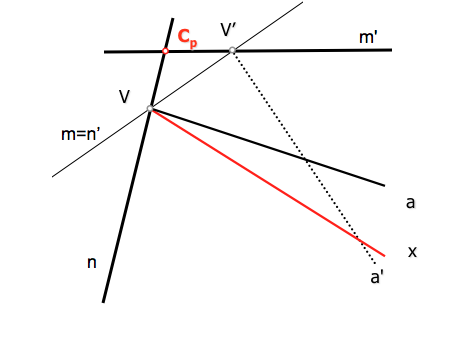If we cut by a’ the line x, generated point (A’) and its counterpart (series basis) will find themselves aligned with the projective center. The homologous point (A) element contains (x’) Searches.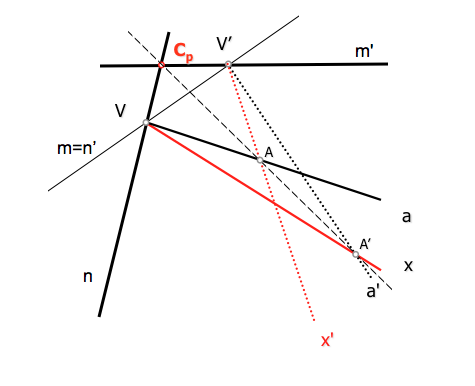### Examples

To complete the study some worked examples that reinforce the concepts proposed.

Determine the projective center of the beams and the homolog of one of the beams in the following cases:

to)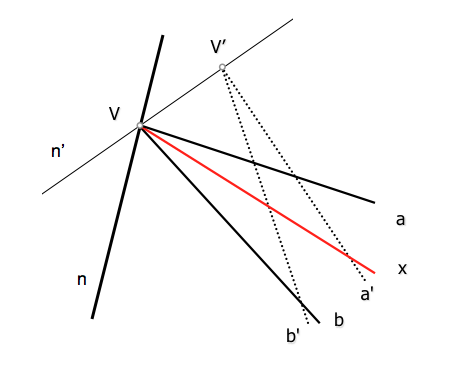b)